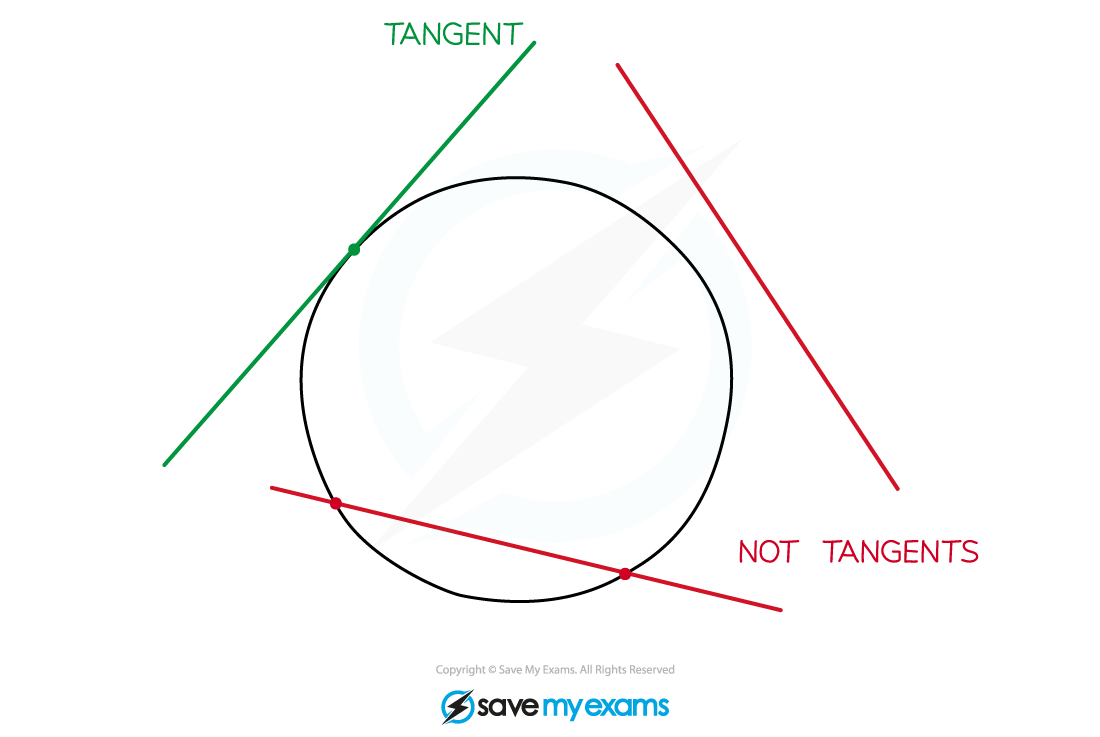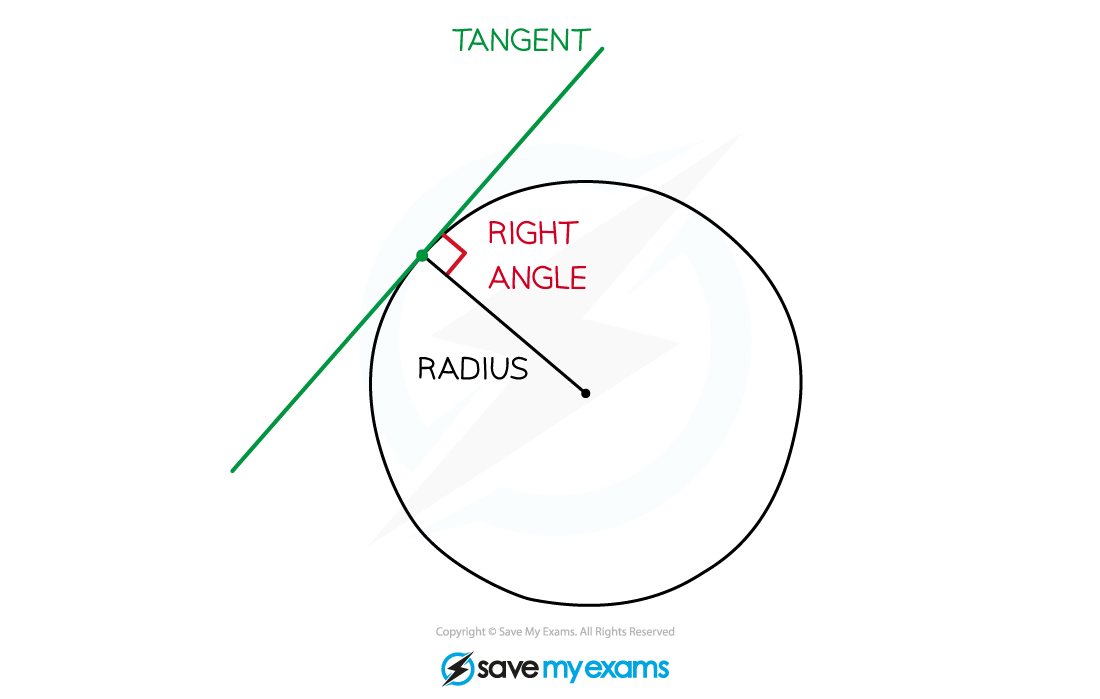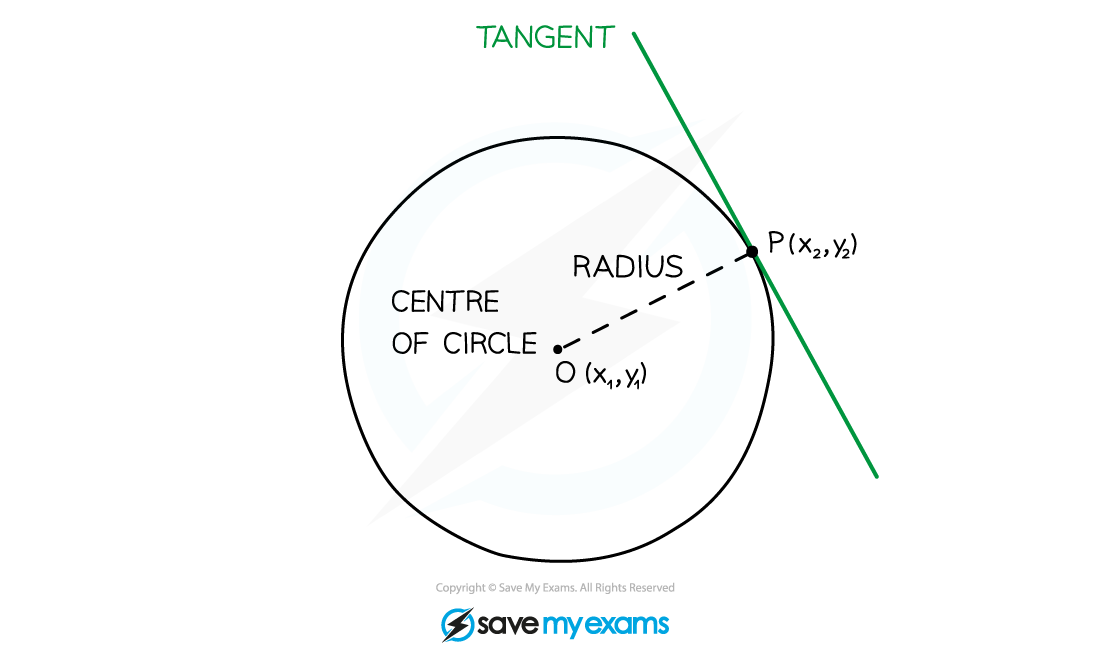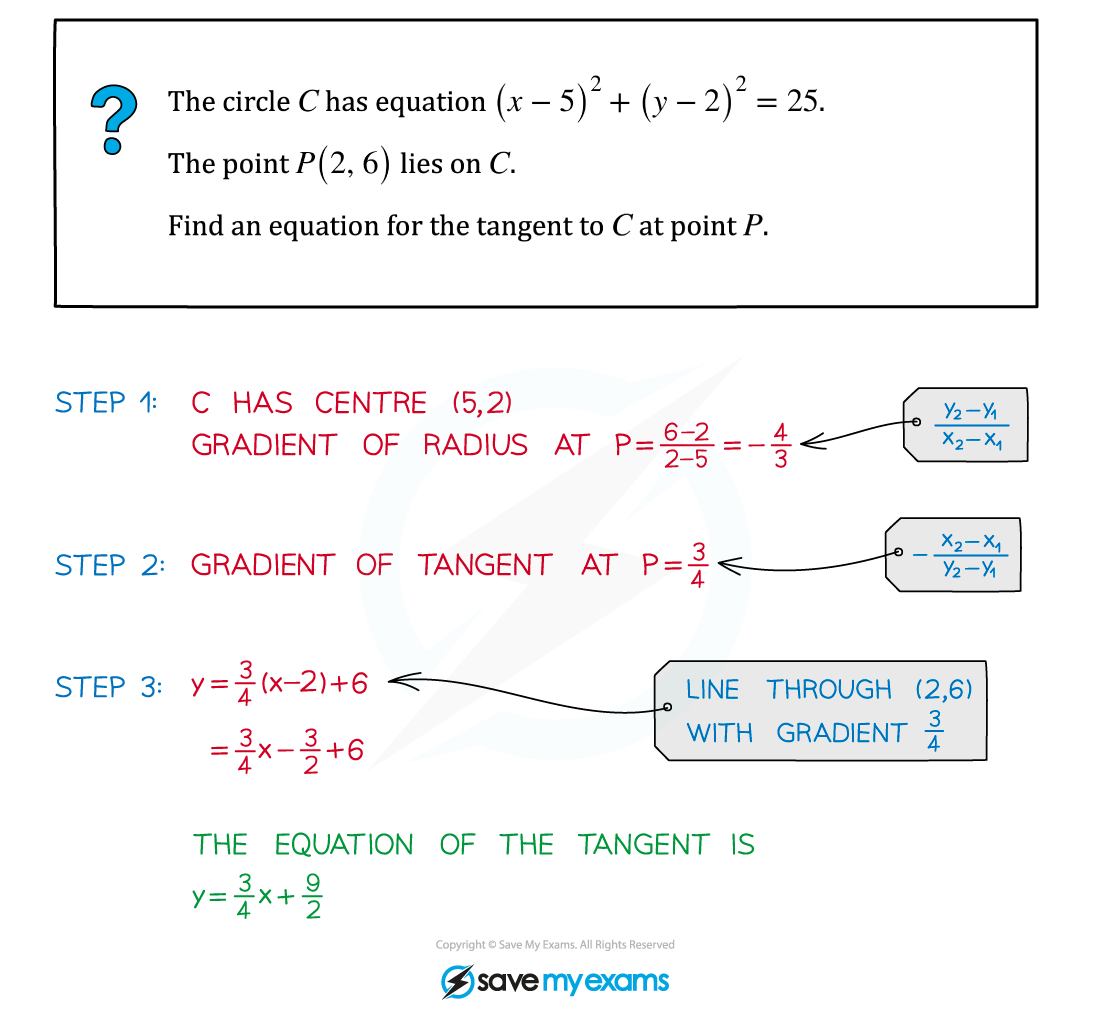# AQA A Level Maths: Pure复习笔记3.2.5 Radius & Tangent

#### What is the relationship between tangents and radii?

• A tangent is a line that touches a circle at a single point but doesn't cut across the circle• A tangent to a circle is perpendicular to the radius of the circle at the point of intersection#### How can I find the equation of the tangent line to a circle at a given point?• STEP 2: Find the gradient of the tangent• STEP 3: The equation of the tangent is the equation of the line with that gradient that goes through point P (see Equation of a Straight Line)

#### Exam Tip

• If you understand the formula in Step 2 above, you can find the gradient of the tangent without having to find the gradient of the radius first.

#### Worked Example